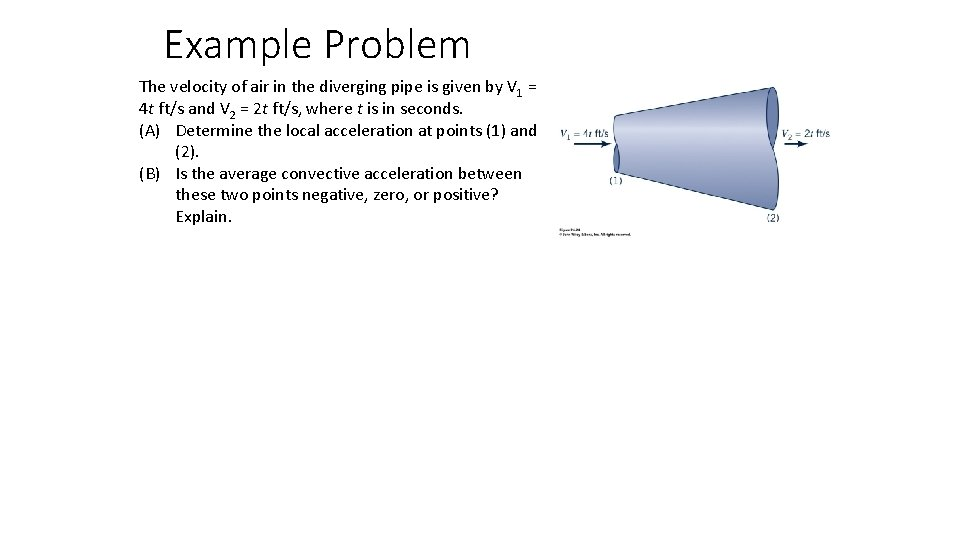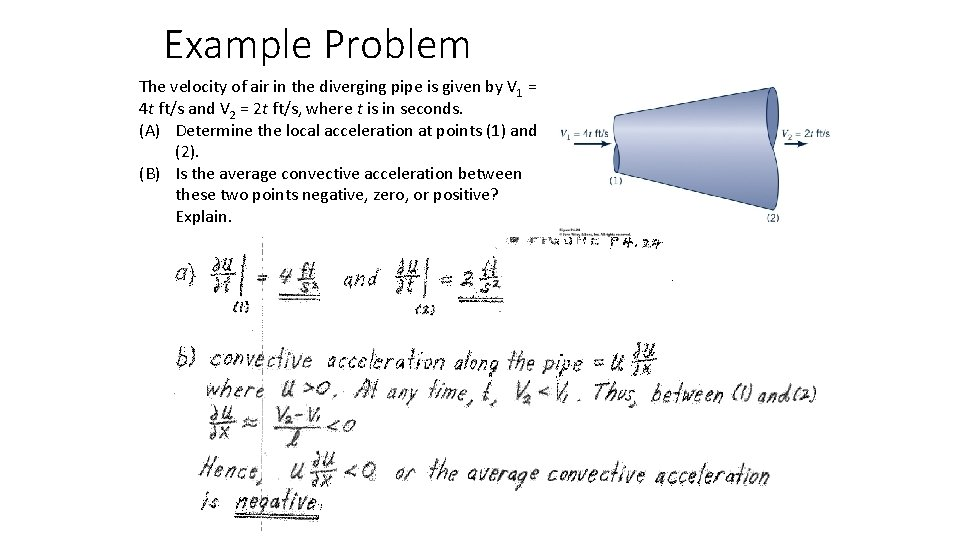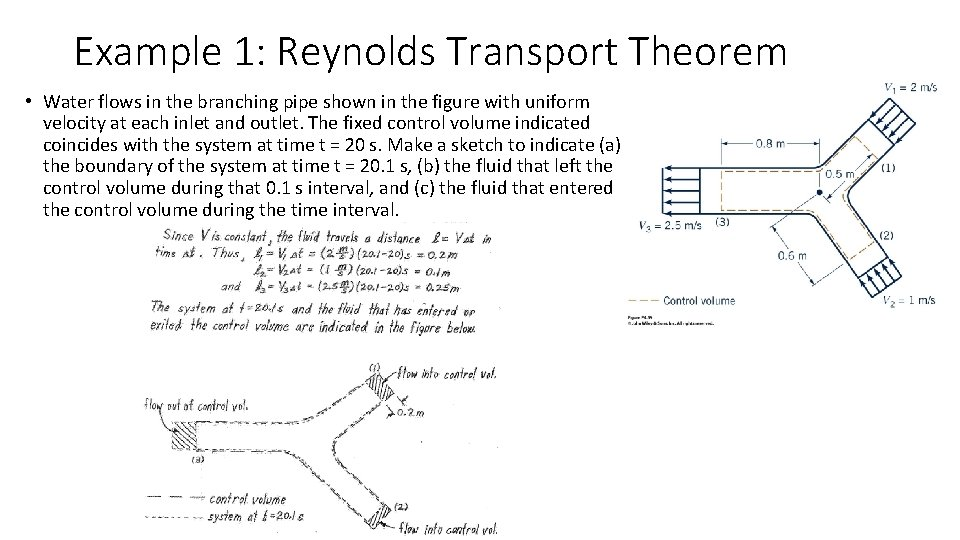# Example Problem The velocity of air in the

• Slides: 6Example Problem The velocity of air in the diverging pipe is given by V 1 = 4 t ft/s and V 2 = 2 t ft/s, where t is in seconds. (A) Determine the local acceleration at points (1) and (2). (B) Is the average convective acceleration between these two points negative, zero, or positive? Explain.Example Problem The velocity of air in the diverging pipe is given by V 1 = 4 t ft/s and V 2 = 2 t ft/s, where t is in seconds. (A) Determine the local acceleration at points (1) and (2). (B) Is the average convective acceleration between these two points negative, zero, or positive? Explain.Example 1: Reynolds Transport Theorem • Water flows in the branching pipe shown in the figure with uniform velocity at each inlet and outlet. The fixed control volume indicated coincides with the system at time t = 20 s. Make a sketch to indicate (a) the boundary of the system at time t = 20. 1 s, (b) the fluid that left the control volume during that 0. 1 s interval, and (c) the fluid that entered the control volume during the time interval.Example 2: Reynolds Transport Theorem • Water flows from a nozzle with a speed of V = 10 m/s and is collected in a container that moves toward the nozzle with a speed of Vcv = 2 m/s, as shown in figure. The moving control surface consists of the inner surface of the container. The system consists of the water in the container at time t = 0 and the water between the nozzle and the tank in the constant diameter stream at t=0. At time t = 0. 1 s what volume of the system remains outside of the control volume?Example 1: Reynolds Transport Theorem • Water flows in the branching pipe shown in the figure with uniform velocity at each inlet and outlet. The fixed control volume indicated coincides with the system at time t = 20 s. Make a sketch to indicate (a) the boundary of the system at time t = 20. 1 s, (b) the fluid that left the control volume during that 0. 1 s interval, and (c) the fluid that entered the control volume during the time interval.Example 2: Reynolds Transport Theorem • Water flows from a nozzle with a speed of V = 10 m/s and is collected in a container that moves toward the nozzle with a speed of Vcv = 2 m/s, as shown in figure. The moving control surface consists of the inner surface of the container. The system consists of the water in the container at time t = 0 and the water between the nozzle and the tank in the constant diameter stream at t=0. At time t = 0. 1 s what volume of the system remains outside of the control volume?